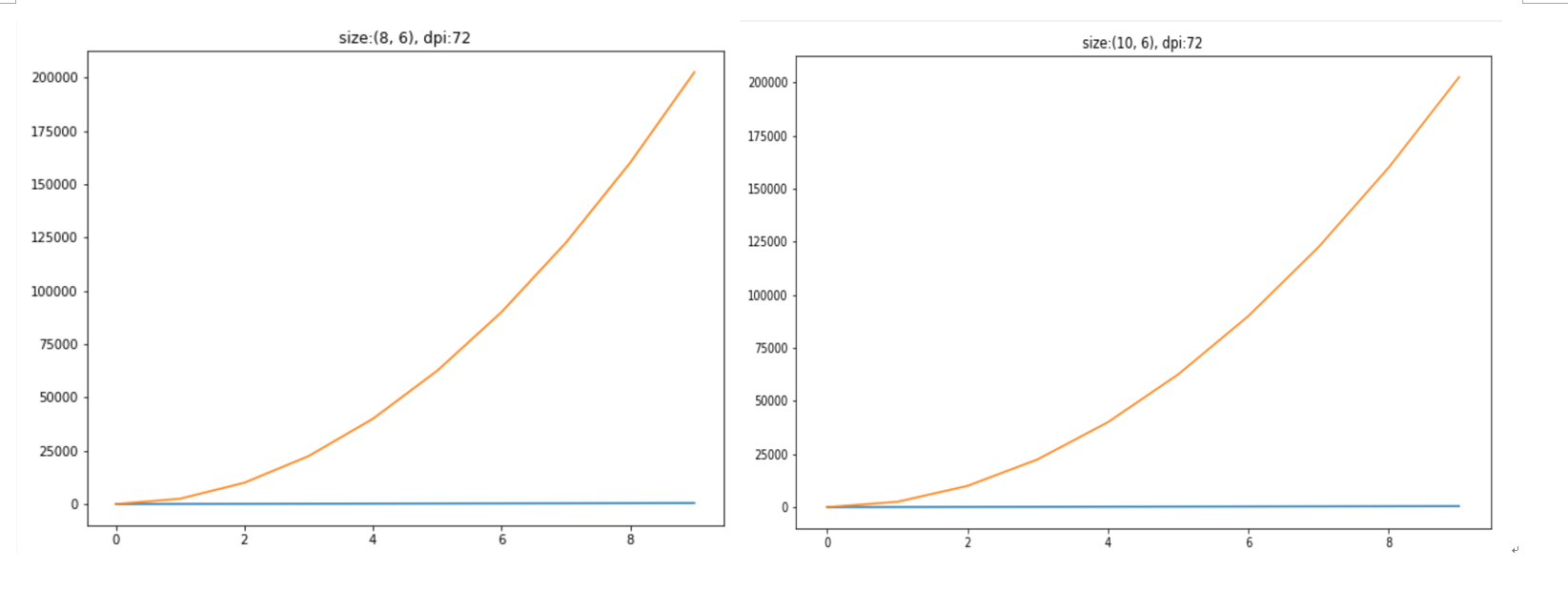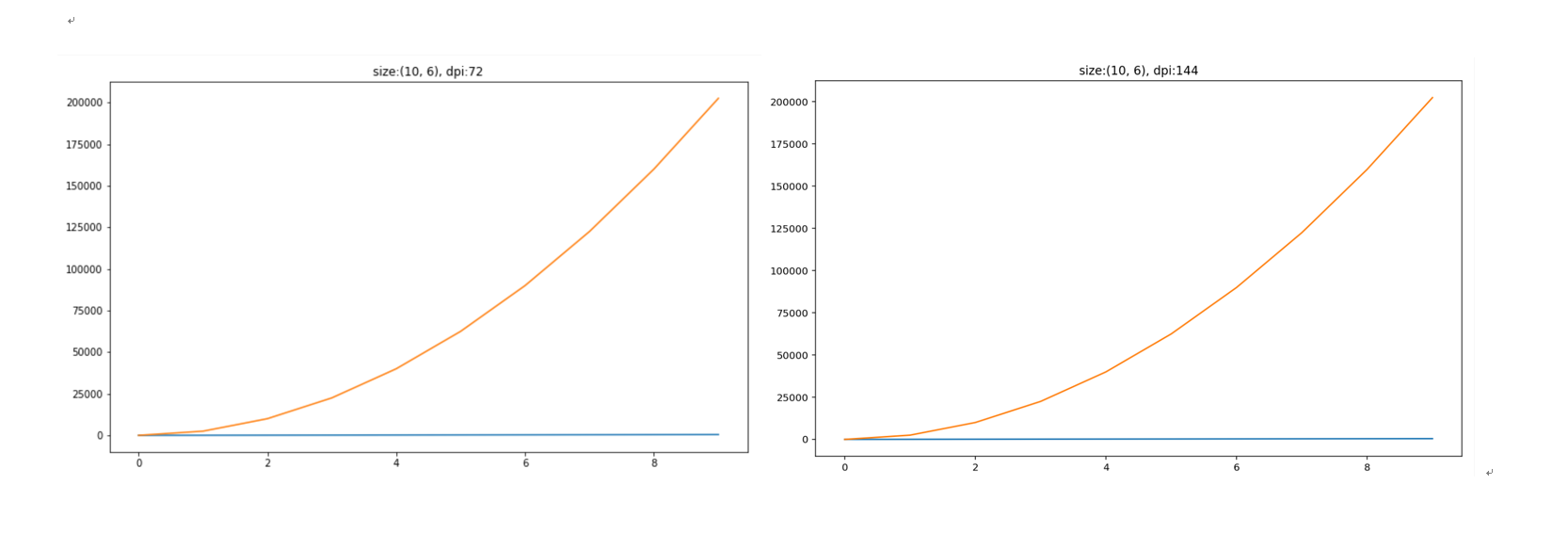# Matplotlib中图像大小设置

2019-10-15 23:53:30 来源: 晴天小雨 0

#### Matplotlib设置图形大小的语句

fig = plt.figure(figsize=(width, height), dpi=dpi)

dpi设置图形每英寸点数(ppi)；

px， py = width * dpi, height  * dpi

#### 同一像素尺寸的图形可以有不同的表示

figsize=(15, 7.5), dpi=80

figsize=(12, 6), dpi=100

figsize=(8, 4), dpi=150

figsize=(6, 3), dpi=200

#### 图中元素的大小ps：(近视的你肯定看不出图片的差距，因为不近视的也看不出啥区别，笑！)

#### 结论

• 图形尺寸（figsize）确定图形的大小（以英寸为单位）。

• dpi 确定了图形每英寸包含的像素数，图形尺寸相同的情况下， dpi 越高，则图像的清晰度越高

#### 附:图形复现代码

import matplotlib.pyplot as plt

if __name__ == '__main__':
data = [(x, x**2) for x in range(0, 500, 50)]
dpi_list = [72, 72, 72, 144]
width_list = [8, 10, 10, 10]
subplot_list = list(range(1, 7))
for width, dpi, in zip(width_list, dpi_list):
fig = plt.figure(figsize=(width, 6), dpi=dpi)
plt.title("size:{}, dpi:{}".format((width, 6), dpi))
plt.grid(True)
plt.plot(data)
plt.show()

0条评论